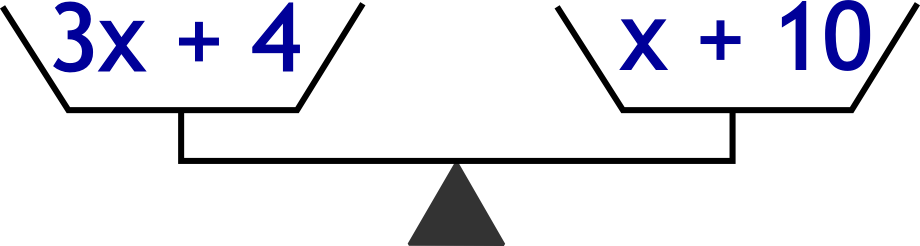The Singing Hedgehog Guide to:
Algebra - Equations 2

Here is a simple example:
Solve the equation 3x + 4 = x + 10

What do we want it to say? We want it to say "x equals the answer"
What's in the wrong place? The plus 4 is clearly wrong
How do we get rid of it? We take it away

Remember that equations are like balances.If we take away 4 from the left we must take away 4 from the right.

next page